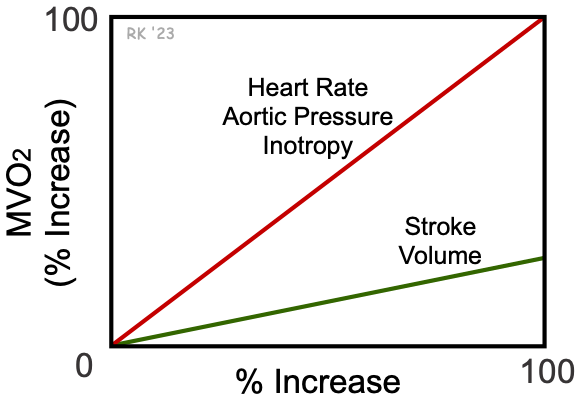# Determinants of Myocardial Oxygen Consumption

Myocyte contraction is the primary factor determining myocardial oxygen consumption (MVO2) above basal levels. Therefore, factors that enhance tension development by the cardiac muscle cells, the rate of tension development, or the number of tension generating cycles per unit time will increase MVO2. For example, doubling the heart rate approximately doubles MVO2 because ventricular myocytes are generating twice the number of tension cycles per minute. Increasing inotropy also increases MVO2 because the rate of tension development is increased as well as the magnitude of tension, both of which result in increased ATP hydrolysis and oxygen consumption. Increasing afterload (e.g., aortic pressure), because it increases myocyte tension development, also increases MVO2. Increasing preload (e.g., ventricular end-diastolic volume) increases MVO2; however, the increase is much less than what might be expected because of the wall stress relationship as described below.

Changes in MVO2 are closely related to changes in ventricular wall stress, which is also used as an index of ventricular afterload. This equation is based on the Law of LaPlace, which states that wall tension (T) is proportionate to the pressure (P) times radius (r) for thin-walled spheres or cylinders. Wall stress is tension divided by wall thickness (h). Therefore, an increase in intraventricular pressure development, or an increase in chamber radius, increases wall stress, whereas an increase in wall thickness (as occurs in hypertrophy) decreases wall stress.

If we assume, for simplicity, that the shape of the ventricular chamber approximates a sphere during contraction, then volume is related to radius by V = 4/3 · r3. Therefore, radius (or diameter) is proportional to the cube root of volume. If we substitute this into the wall stress equation, then we have the following relationship:This relationship shows that a 100% increase in ventricular volume increases wall stress by only 26%. In contrast, increasing intraventricular pressure by 100% increases wall stress by 100%. For this reason, wall stress, and therefore MVO2, is far less sensitive to changes in ventricular volume than pressure (see figure at right). In summary, increasing heart rate, aortic pressure, and inotropy increase MVO2 about 4-times more than an equivalent percent increase in stroke volume (SV).